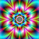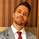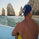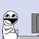# Ehlers Smoothed Stochastic & RSI with Roofing Filters

‎16071‎ مشاهدة
16071
Roofing filters, first discussed by Mr.John Ehlers , act as a passband, filtering out unwanted noise from market data and accentuating turning points.

I have included 2 indicators with filters enabled. Both support double smoothing via options page. All the parameters are configurable.

Info on Roofing Filter and Ehlers Super Smoother:
----------------------------------------------------
The Ehlers' Roofing Filter is an expansion on Ehlers Super Smoother Filter, both being smoothing techniques based on analog filters. This filter aims at reducing noise in price data.

In Super Smoother Filter, regardless of the time frame used, all waves having cycles of less than 10 bars are considered noise (customizable via options page). The Roofing Filter uses this principle, however, it also creates a so-called "roof" by eliminating wave components having cycles greater than 48 bars which are perceived as "spectral dilation". Thus, the filter only passes those spectral components whose periods are between 10 and 48 bars. This technique noticeably reduces indicator lag and also helps assess turning points more accurately.

- Spectral dilation paper: http://www.mesasoftware.com/Seminars/Spe...

------------------------------------------------------
If you want to use RSI %B and Bandwidth, follow this guide to "Make mine" this chart and get access to the source:

For the complete list of my indicators, check this post:
```//
// @author LazyBear
// List of all my indicators: https://www.tradingview.com/v/4IneGo8h/
//
study("Ehlers Smoothed Stochastic [LazyBear]", shorttitle="ESSTOCH_LB")
PI=3.14159265359
applyDoubleSmoothing=input(false, type=bool)
length = input (14, title="Stoch Length")
lengthMA=input (3, title="Stoch MA Length")
over_bought = input (.8)
over_sold = input (.2)
src=close
roofingBandUpper=input(48)
roofingBandLower=input(10)

a1 = exp(-PI * sqrt(2) / lower)
coeff2 = 2 * a1 * cos(sqrt(2) * PI / lower)
coeff3 = - pow(a1,2)
coeff1 = 1 - coeff2 - coeff3
filt = coeff1 * (price + nz(price)) / 2 + coeff2 * nz(filt) + coeff3 * nz(filt)
filt

EhlersRoofingFilter(price, smoothed, upper, lower) =>
alpha1 = (cos(sqrt(2) * PI / upper) + sin (sqrt(2) * PI / upper) - 1) / cos(sqrt(2) * PI / upper)
highpass = pow(1 - alpha1 / 2, 2) * (price - 2 * nz(price) + nz(price)) +
2 * (1 - alpha1) * nz(highpass) - pow(1 - alpha1, 2) * nz(highpass)
smoothed ? EhlersSuperSmootherFilter(highpass, lower) : highpass

EhlersStochastic(price, length, applyEhlerSmoothing, roofingBandUpper, roofingBandLower) =>
filt = EhlersRoofingFilter(price, applyEhlerSmoothing, roofingBandUpper, roofingBandLower)
highestP = highest(filt, length)
lowestP = lowest(filt, length)
iff ((highestP - lowestP) != 0, (filt - lowestP) / (highestP - lowestP),  0)

hline (over_bought)
hline (over_sold)
hline((over_bought+over_sold)/2)
plot(sma(stoch, lengthMA), color=red, linewidth=1)
plot(stoch, color=blue, linewidth=1)

```Выложите пожалуйста индикатор RSI with Roofing Filters, его нигде нет.
Спасибо!)
ردThe second function

alpha1 = (cos(sqrt(2) * PI / upper) + sin (sqrt(2) * PI / upper) - 1) / cos(sqrt(2) * PI / upper)

should be

alpha1 = (cos(sqrt(2) * PI / (2 * upper)) + sin (sqrt(2) * PI / (2 * upper)) - 1) / cos(sqrt(2) * PI / (2 * upper))

Ehlers used 0.707 in his calculations for the spectral dilation ( = sqrt(2) / 2) so you are missing a '/2' somewhere in the multiplication chain of each trig function argument.
ردIn the first function:

coeff2 = 2 * a1 * cos(sqrt(2) * PI / lower)

should be

coeff2 = 2 * a1 * cos(sqrt(2) * PI / l(2 * lower))

Ehlers multiplied by 180 degrees in his formula (PI / 2), but pine uses radians so everything else is correct.
ردmortdiggiddy
@mortdiggiddy, sorry:

coeff2 = 2 * a1 * cos(sqrt(2) * PI / (2 * lower))
ردi cant seem to get the RSI to load on my charts and the script seems to only have the stoch ?
ردwith double smoothing turned on… Wow.
Thanks, LB,
ردgrahvity
yw.

Try comparing this to normal RSI, you will see how much filtering helps :)
رد